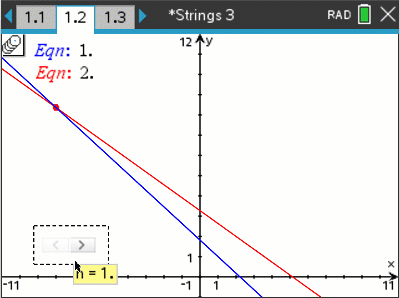# Activities

•• ##### Author60 Minutes

• ##### Device
•TI-Nspire™ CX CAS
• TI-Nspire™ CAS
• ##### Software

TI-Nspire™ CAS

5.0

## String Graphs Part3#### Activity Overview

This is part three of a four part series. In this activity students find the points of intersection between consecutive straight lines. (Simultaneous Equations). Students use a range of techniques: by-hand, graphically and using CAS. Students then formulate equations to summaries the consecutive points of intersection.

#### Objectives

Students use a range of techniques to solve pairs of simultaneous equations in order to determine the consecutive points of intersection for the lines generated in activity two of this series. The techniques include by-hand, simulated by-hand and using the solve command on their calculator. Students observe the patterns in the consecutive points of intersection and connect the dots by establishing an equation.

#### Vocabulary

• Simultaneous Equations
• Consecutive
• Parameter
• Family of straight lines